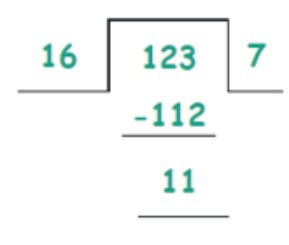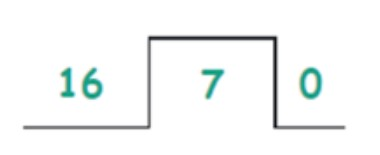# Decimal to Hex Converter

Type or paste the hex decimal number in the input box to convert it into hexadecimal number form using this decimal to hex converter.

Error: UnKnown number
Swap

Give Us Feedback

Decimal to hex converter is used to convert the decimal numbers into a hexadecimal number system. This converter uses positive and negative decimal numbers for the conversion of decimal to hex.

## What is a decimal number system?

The decimal number system also called base 10 numbers are the numerical digits from zero to nine. For example, 3452 is a decimal number.

• In this number, 2 is at one’s place (2^0 is 1)
• 5 is in tenth place (10^1)
• 4 is at the hundredth place (10^2)
• 3 is at the thousandth place (10^3)

## What is a hexadecimal number system?

The base 16 numbers are known as the hexadecimal number system. Due to base 16, this system has numerals from 0 to 15. To address a two-digit number, the alphabets A, B, C, D, E, and F are used.

• A is used for 10
• B is used for 11
• C is used for 12
• D is used for 13
• E is used for 14
• F is used for 15

## How to convert decimal to hex?

Following is a solved example of the conversion of decimal to hex.

Example

Convert 123 from decimal to hex.

Solution

Step 1: Divide the given decimal number by 16.

123/16Step 2: The remainder will be the rightmost digit of a hexadecimal number.

11 = B is the rightmost digit.

Step 3: Divide the quotient by 16 and repeat the process until it gives zero.It gives zero as the quotient because 7 is less than 16. So, 7 will be the remainder.

Hence the hexadecimal number of 123 is 7B.

### Math Tools